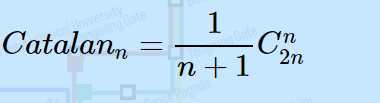# 复旦大学研究生机试（2019）

## 1. 计算机学院

### 1.1 相隔天数

20190208

3

##### 解题：

*闰年：*当年数不能被100整除时，但若能被4整除则为闰年，或者其能被400整除也是闰年。

#include <iostream>
#include <stdio.h>
#include <string>
#include <sstream>
using namespace std;

bool isrun(int year){
if((year%100!=0&&year%4==0)||(year%400==0))
return true;
else
return false;
}

int main()
{
string str;
int year, month, day,countday = 0;
scanf("%4d%2d%2d",&year,&month,&day);
int mdays[]={31,28,31,30,31,30,31,31,30,31,30,31};
//统一到同一年
while(year>2019){
year--;
if(isrun(year))
countday+=366;
else
countday+=365;
}
while(year<2019){
if(isrun(year))
countday-=366;
else
countday-=365;
year++;
}
//统一到同一月
while(month>2){
month--;
if(month!=2){
countday += mdays[month-1];
}else if(isrun(year)){
countday+=29;
}else{
countday+=28;
}
}
while(month<2){
if(month!=2){
countday -= mdays[month-1];
}else if(isrun(year)){
countday-=29;
}else{
countday-=28;
}
month++;
}
//计算同一月的日期差
countday+=day-5;
cout<<countday<<endl;
return 0;
}



### 1.2 最大连续子序列

##### 题目：

6
-2 11 -4 13 -5 -2

20

##### 解题：

#include <iostream>
#include <stdio.h>
#include <vector>
#include <limits.h>
using namespace std;

int main()
{
int N;
scanf("%d",&N);
vector<int> vec;
while(N-->0){
int temp;
scanf("%d",&temp);
vec.push_back(temp);
}
int maxa = INT_MIN;
for(int i =0;i<vec.size();i++){
int currSum = 0;
for(int j = i;j<vec.size();j++){
currSum+=vec[j];
if(currSum>maxa)
maxa = currSum;
}
}
cout<<maxa<<endl;
return 0;
}



https://www.cnblogs.com/conw/p/5896155.html

### 1.3 有向树形态

3

5

##### 求解：#include <iostream>
using namespace std;

long long Cmn(long long n) {
long long fengzi = 1, fengmu = 1;
for (int i = 2 * n; i > n; i--) {
fengzi *= i;
}
for (int i = n; i > 1; i--) {
fengmu *= i;
}
return fengzi / fengmu;
}

int main() {
long long N;
cin >> N;
cout << Cmn(N) / (N + 1) << endl;
return 0;
}


import java.math.BigInteger;
import java.util.Scanner;
public class Main {
public static BigInteger Cmn(int n){
BigInteger fengzi = new BigInteger("1"),fengmu = new BigInteger("1");
for (int i = 2 * n; i > n; i--) {
fengzi = fengzi.multiply(new BigInteger(Integer.toString(i)));
}
for (int i = n; i > 1; i--) {
fengmu = fengmu.multiply(new BigInteger(Integer.toString(i)));
}
return fengzi.divide(fengmu);
}
public static void main(String[] args) {
// TODO Auto-generated method stub
Scanner sc = new Scanner(System.in);
int N = sc.nextInt();
System.out.println(Cmn(N).divide(new BigInteger(Integer.toString(N+1))).toString());
}
}



def Cmn(n):
fengzi=int(1)
fengmu=int(1)
for i in range(n+1,2*n+1):
fengzi *= i
for i in range(2, n+1):
fengmu *= i
return fengzi / fengmu

N = int(input())
print(int(Cmn(N)/(N+1)))


## 2. 工研院

### 2.1 手机按键

##### 题目：

[ 1 ] [ 2ABC ] [ 3DEF  ]
[ 4GHI  ] [ 5JKL ] [ 6MNO  ]
[ 7PQRS ] [ 8TUV ] [ 9WXYZ ]
[ 0空  ]


255

AK

2222------55

AK

5
1 2 2 3 1
2
1 2 5
2 5 -1

6 6 1 2 0

### 2.3 计算通讯代价

##### 题目：

1————2————3


3
1 2
2 3

3 2 303-07711
05-193955
06-03754
03-23339
05-273954
05-13183
03-154622
04-2977
06-30
01-22480
02-21
06-031309
04-06206
03-053449
02-0476
02-0598
©️2020 CSDN 皮肤主题: 像素格子 设计师:CSDN官方博客